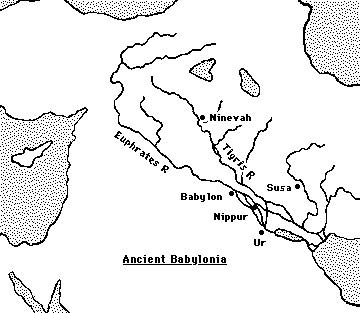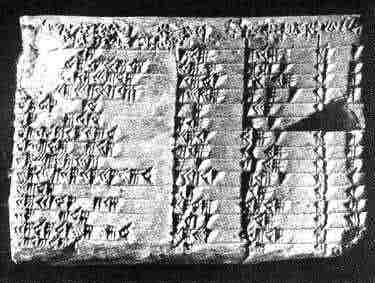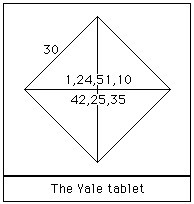# Pythagoras's theorem in Babylonian mathematics

Pythagoras's theorem in Babylonian mathematics

In this article we examine four Babylonian tablets which all have some connection with Pythagoras's theorem. Certainly the Babylonians were familiar with Pythagoras's theorem. A translation of a Babylonian tablet which is preserved in the British museum goes as follows:-
4 is the length and 5 the diagonal. What is the breadth ?
Its size is not known.
4 times 4 is 16.
5 times 5 is 25.
You take
16 from 25 and there remains 9.
What times what shall I take in order to get
9 ?
3 times 3 is 9.
All the tablets we wish to consider in detail come from roughly the same period, namely that of the Old Babylonian Empire which flourished in Mesopotamia between 1900 BC and 1600 BC.Here is a map of the region where the Babylonian civilisation flourished.

The article Babylonian mathematics gives some background to how the civilisation came about and the mathematical background which they inherited.

The four tablets which interest us here we will call the Yale tablet YBC 7289, Plimpton 322 (shown below), the Susa tablet, and the Tell Dhibayi tablet. Let us say a little about these tablets before describing the mathematics which they contain.

The Yale tablet YBC 7289 which we describe is one of a large collection of tablets held in the Yale Babylonian collection of Yale University. It consists of a tablet on which a diagram appears. The diagram is a square of side 30 with the diagonals drawn in. The tablet and its significance was first discussed in  and recently in .Plimpton 322 is the tablet numbered 322 in the collection of G A Plimpton housed in Columbia University.

You can see from the picture that the top left hand corner of the tablet is damaged as and there is a large chip out of the tablet around the middle of the right hand side. Its date is not known accurately but it is put at between 1800 BC and 1650 BC. It is thought to be only part of a larger tablet, the remainder of which has been destroyed, and at first it was thought, as many such tablets are, to be a record of commercial transactions. However in  Neugebauer and Sachs gave a new interpretation and since then it has been the subject of a huge amount of interest.

The Susa tablet was discovered at the present town of Shush in the Khuzistan region of Iran. The town is about 350 km from the ancient city of Babylon. W K Loftus identified this as an important archaeological site as early as 1850 but excavations were not carried out until much later. The particular tablet which interests us here investigates how to calculate the radius of a circle through the vertices of an isosceles triangle.

Finally the Tell Dhibayi tablet was one of about 500 tablets found near Baghdad by archaeologists in 1962. Most relate to the administration of an ancient city which flourished in the time of Ibalpiel II of Eshunna and date from around 1750. The particular tablet which concerns us is not one relating to administration but one which presents a geometrical problem which asks for the dimensions of a rectangle whose area and diagonal are known.

Before looking at the mathematics contained in these four tablets we should say a little about their significance in understanding the scope of Babylonian mathematics. Firstly we should be careful not to read into early mathematics ideas which we can see clearly today yet which were never in the mind of the author. Conversely we must be careful not to underestimate the significance of the mathematics just because it has been produced by mathematicians who thought very differently from today's mathematicians. As a final comment on what these four tablets tell us of Babylonian mathematics we must be careful to realise that almost all of the mathematical achievements of the Babylonians, even if they were all recorded on clay tablets, will have been lost and even if these four may be seen as especially important among those surviving they may not represent the best of Babylonian mathematics.

There is no problem understanding what the Yale tablet YBC 7289 is about.Here is a Diagram of Yale tablet

It has on it a diagram of a square with 30 on one side, the diagonals are drawn in and near the centre is written 1,24,51,10 and 42,25,35. Of course these numbers are written in Babylonian numerals to base 60. See our article on Babylonian numerals. Now the Babylonian numbers are always ambiguous and no indication occurs as to where the integer part ends and the fractional part begins. Assuming that the first number is 1; 24,51,10 then converting this to a decimal gives 1.414212963 while √2 = 1.414213562. Calculating 30 × [ 1;24,51,10 ] gives 42;25,35 which is the second number. The diagonal of a square of side 30 is found by multiplying 30 by the approximation to √2.

This shows a nice understanding of Pythagoras's theorem. However, even more significant is the question how the Babylonians found this remarkably good approximation to √2. Several authors, for example see  and , conjecture that the Babylonians used a method equivalent to Heron's method. The suggestion is that they started with a guess, say x. They then found e = x2 - 2 which is the error. Then
(x - e/2x)2 = x2 - e + (e/2x)2 = 2 + (e/2x)2
and they had a better approximation since if e is small then (e/2x)2 will be very small. Continuing the process with this better approximation to √2 yieds a still better approximation and so on. In fact as Joseph points out in , one needs only two steps of the algorithm if one starts with x = 1 to obtain the approximation 1;24,51,10.

This is certainly possible and the Babylonians' understanding of quadratics adds some weight to the claim. However there is no evidence of the algorithm being used in any other cases and its use here must remain no more than a fairly remote possibility. May I [EFR] suggest an alternative. The Babylonians produced tables of squares, in fact their whole understanding of multiplication was built round squares, so perhaps a more obvious approach for them would have been to make two guesses, one high and one low say a and b. Take their average (a + b)/2 and square it. If the square is greater than 2 then replace b by this better bound, while if the square is less than 2 then replace a by (a + b)/2. Continue with the algorithm.

Now this certainly takes many more steps to reach the sexagesimal approximation 1;24,51,10. In fact starting with a = 1 and b = 2 it takes 19 steps as the table below shows:
```step        decimal           sexagesimal

1        1.500000000        1;29,59,59
2        1.250000000        1;14,59,59
3        1.375000000        1;22,29,59
4        1.437500000        1;26,14,59
5        1.406250000        1;24,22,29
6        1.421875000        1;25,18,44
7        1.414062500        1;24,50,37
8        1.417968750        1;25, 4,41
9        1.416015625        1;24,57,39
10        1.415039063        1;24,54, 8
11        1.414550781        1;24,52,22
12        1.414306641        1;24,51;30
13        1.414184570        1;24,51; 3
14        1.414245605        1;24,51;17
15        1.414215088        1;24,51;10
16        1.414199829        1;24,51; 7
17        1.414207458        1;24,51; 8
18        1.414211273        1;24,51; 9
19        1.414213181        1;24,51;10
```
However, the Babylonians were not frightened of computing and they may have been prepared to continue this straightforward calculation until the answer was correct to the third sexagesimal place.Next we look again at Plimpton 322

The tablet has four columns with 15 rows. The last column is the simplest to understand for it gives the row number and so contains 1, 2, 3, ... , 15. The remarkable fact which Neugebauer and Sachs pointed out in  is that in every row the square of the number c in column 3 minus the square of the number b in column 2 is a perfect square, say h.
c2 - b2 = h2
So the table is a list of Pythagorean integer triples. Now this is not quite true since Neugebauer and Sachs believe that the scribe made four transcription errors, two in each column and this interpretation is required to make the rule work. The errors are readily seen to be genuine errors, however, for example 8,1 has been copied by the scribe as 9,1.

The first column is harder to understand, particularly since damage to the tablet means that part of it is missing. However, using the above notation, it is seen that the first column is just (c/h)2. Now so far so good, but if one were writing down Pythagorean triples one would find much easier ones than those which appear in the table. For example the Pythagorean triple 3, 4 , 5 does not appear neither does 5, 12, 13 and in fact the smallest Pythagorean triple which does appear is 45, 60, 75 (15 times 3, 4 , 5). Also the rows do not appear in any logical order except that the numbers in column 1 decrease regularly. The puzzle then is how the numbers were found and why are these particular Pythagorean triples are given in the table.

Several historians (see for example ) have suggested that column 1 is connected with the secant function. However, as Joseph comments :-
This interpretation is a trifle fanciful.
Zeeman has made a fascinating observation. He has pointed out that if the Babylonians used the formulas h = 2mn, b = m2-n2, c = m2+n2 to generate Pythagorean triples then there are exactly 16 triples satisfying n ≤ 60, 30° ≤ t ≤ 45°, and tan2t = h2/b2 having a finite sexagesimal expansion (which is equivalent to m, n, b having 2, 3, and 5 as their only prime divisors). Now 15 of the 16 Pythagorean triples satisfying Zeeman's conditions appear in Plimpton 322. Is it the earliest known mathematical classification theorem? Although I cannot believe that Zeeman has it quite right, I do feel that his explanation must be on the right track.

To give a fair discussion of Plimpton 322 we should add that not all historians agree that this tablet concerns Pythagorean triples. For example Exarchakos, in , claims that the tablet is connected with the solution of quadratic equations and has nothing to do with Pythagorean triples:-
... we prove that in this tablet there is no evidence whatsoever that the Babylonians knew the Pythagorean theorem and the Pythagorean triads.
I feel that the arguments are weak, particularly since there are numerous tablets which show that the Babylonians of this period had a good understanding of Pythagoras's theorem. Other authors, although accepting that Plimpton 322 is a collection of Pythagorean triples, have argued that they had, as Viola writes in , a practical use in giving a:-
... general method for the approximate computation of areas of triangles.
The Susa tablet sets out a problem about an isosceles triangle with sides 50, 50 and 60. The problem is to find the radius of the circle through the three vertices.Here is a Diagram of Susa tablet

Here we have labelled the triangle A, B, C and the centre of the circle is O. The perpendicular AD is drawn from A to meet the side BC. Now the triangle ABD is a right angled triangle so, using Pythagoras's theorem AD2 = AB2 - BD2, so AD = 40. Let the radius of the circle by x. Then AO = OB = x and OD = 40 - x. Using Pythagoras's theorem again on the triangle OBD we have
x2 = OD2 + DB2.
So
x2 = (40-x)2 + 302
giving x2 = 402 - 80x + x2 + 302

and so 80x = 2500 or, in sexagesimal, x = 31;15.

Finally consider the problem from the Tell Dhibayi tablet. It asks for the sides of a rectangle whose area is 0;45 and whose diagonal is 1;15. Now this to us is quite an easy exercise in solving equations. If the sides are x, y we have xy = 0.75 and x2 + y2 = (1.25)2. We would substitute y = 0.75/x into the second equation to obtain a quadratic in x2 which is easily solved. This however is not the method of solution given by the Babylonians and really that is not surprising since it rests heavily on our algebraic understanding of equations. The way the Tell Dhibayi tablet solves the problem is, I would suggest, actually much more interesting than the modern method.

Here is the method from the Tell Dhibayi tablet. We preserve the modern notation x and y as each step for clarity but we do the calculations in sexagesimal notation (as of course does the tablet).
Compute 2xy = 1;30.

Subtract from x2 + y2 = 1;33,45 to get x2 + y2 - 2xy = 0;3,45.

Take the square root to obtain x - y = 0;15.

Divide by 2 to get (x - y)/2 = 0;7,30.

Divide x2 + y2 - 2xy = 0;3,45 by 4 to get x2/4 + y2/4 - xy/2 = 0;0,56,15.

Add xy = 0;45 to get x2/4 + y2/4 + xy/2 = 0;45,56,15.

Take the square root to obtain (x + y)/2 = 0;52,30.

Add (x + y)/2 = 0;52,30 to (x - y)/2 = 0;7,30 to get x = 1.

Subtract (x - y)/2 = 0;7,30 from (x + y)/2 = 0;52,30 to get y = 0;45.

Hence the rectangle has sides x = 1 and y = 0;45.
Is this not a beautiful piece of mathematics! Remember that it is 3750 years old. We should be grateful to the Babylonians for recording this little masterpiece on tablets of clay for us to appreciate today.

Article by: J J O'Connor and E F Robertson

List of References (31 books/articles)

 JOC/EFR © December 2000 Copyright information School of Mathematics and Statistics University of St Andrews, Scotland